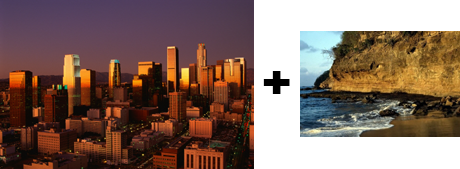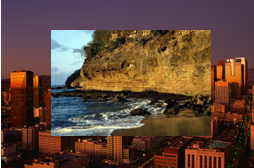﻿ CombineCommand Class | Leadtools.ImageProcessing.Effects | Raster, Medical, Document Help
←Select platform
In This Topic ▼

# CombineCommand Class

Summary
Combines image data from two images (source and destination), letting you specify the areas to be combined, the operations to be performed when combining the data, and which color planes (R or G or B or R, G, and B) are used.
Syntax
C#
Objective-C
C++/CLI
Java
Python
``public class CombineCommand : RasterCommand ``
``@interface LTCombineCommand : LTRasterCommand ``
````public class CombineCommand `
`    extends RasterCommand ````
``public ref class CombineCommand : public RasterCommand   ``
``class CombineCommand(RasterCommand): ``
Remarks
• For example, suppose you use the SpatialFilterCommand to apply an edge detection filter. You can then use this command to combine the resulting image with the original one to produce an image with hard edges.
• This method combines the data byte-by-byte. The two images can be any color resolution. Images that are 24-bits per pixel are the easiest and fastest to combine. This command uses Windows-style coordinates (with a top-left origin) to specify the areas to be combined.
• If the channel setting for one of the images is the master channel (CombineCommandFlags.SourceMaster, CombineCommandFlags.DestinationMaster, or CombineCommandFlags.ResultMaster) the settings for all the images will be set to master.

### Calculating Master Channel Values

In order to speed up widely used image processing filters in LEADTOOLS, the grayscale value (master channel) of a colored image is calculated using the following formulas:

````#define CalcGrayValue(r, g, b) ((L_UCHAR)(((L_UCHAR) (((2 * (L_UINT) (r)) + (5 * (L_UINT) (g)) + (L_UINT) (b) + 4) / 8)))) `
`#define CalcGrayValue16(r, g, b) ((L_UINT16) (((2 * (L_UINT32) (r)) + (5 * (L_UINT32) (g)) + (L_UINT32) (b) + 4) / 8)) `
`#define CalcGrayValue32(r, g, b) ((L_UINT32) (((2 * (L_UINT32) (r)) + (5 * (L_UINT32) (g)) + (L_UINT32) (b) + 4) / 8)) ````
• If a region is defined for either the source or destination image, or both images, the combine applies only to the intersection of regions.
• For example, assume CombineCommandFlags.SourceRed is passed for the source image, CombineCommandFlags.DestinationBlue is passed for the destination image and CombineCommandFlags.ResultGreen is passed for the resulting image. In this case, the green channel of the resulting image is calculated as the result of the operations applied to the red channel of the source image and the blue channel of the destination image. The other channels of the resulting image are unchanged.
• The DestinationRectangle must be defined in order to show the CombineCommand results, because the destination rectangle default values are (0, 0, 0, 0).
• The output image, which is the image that contains the result of the CombineCommand, is set through the Run method. This image is referred to as destination image, result image, or output image.
• The CombineCommand works by combining two images, the source image represented in SourceImage and the destination image, the one that the user sends through the Run method.
• This command supports 12 and 16-bit grayscale and 48 and 64-bit color images. Support for 12 and 16-bit grayscale and 48 and 64-bit color images is available only in the Document/Medical toolkits.
• This command does not support 32-bit grayscale images.
• All images must be either signed or unsigned.

Combine Function - BeforeCombine Function - AfterView additional platform support for this Combine function.

Example

Run the CombineCommand on an image.

C#
````using Leadtools; `
`using Leadtools.Codecs; `
`using Leadtools.ImageProcessing.Effects; `
` `
` `
`public void CombineCommandExample() `
`{ `
`   // Load an image `
`   RasterCodecs codecs = new RasterCodecs(); `
`   codecs.ThrowExceptionsOnInvalidImages = true; `
` `
`   RasterImage image = codecs.Load(Path.Combine(LEAD_VARS.ImagesDir, @"ImageProcessingDemo\Image3.cmp")); `
` `
`   // Prepare the command `
`   CombineCommand command = new CombineCommand(); `
`   command.SourceImage = image.Clone(); `
`   // the rectangle that represents the affected area of the destination image. `
`   command.DestinationRectangle = new LeadRect(image.Width / 8, image.Height / 8, image.Width, image.Height); `
`   // The source point, which represents the source point of the source image which is to be combined. `
`   command.SourcePoint = new LeadPoint(0, 0); `
`   // the operations that will be performed to produce the result, and the channel that will be used to achieve this result. `
`   command.Flags = CombineCommandFlags.OperationAdd | CombineCommandFlags.Destination0 | CombineCommandFlags.SourceRed | CombineCommandFlags.DestinationGreen | CombineCommandFlags.ResultBlue; `
`   command.Run(image); `
`   codecs.Save(image, Path.Combine(LEAD_VARS.ImagesDir, "Result.jpg"), RasterImageFormat.Jpeg, 24); `
` `
`} `
` `
`static class LEAD_VARS `
`{ `
`   public const string ImagesDir = @"C:\LEADTOOLS22\Resources\Images"; `
`} ````
Requirements